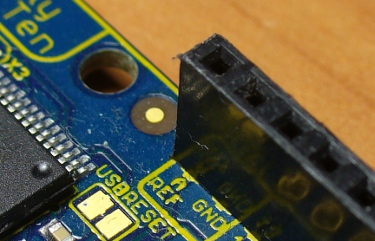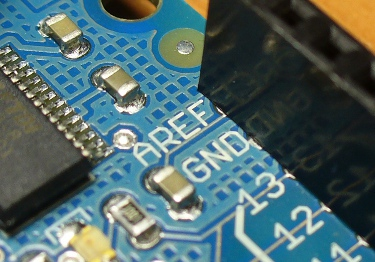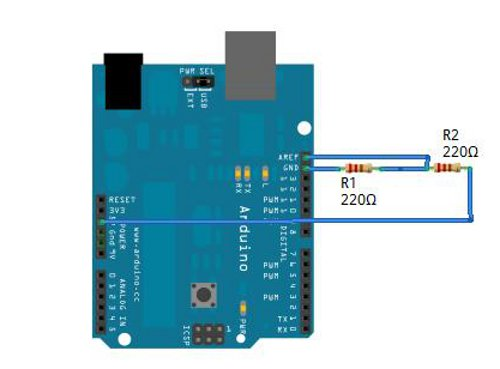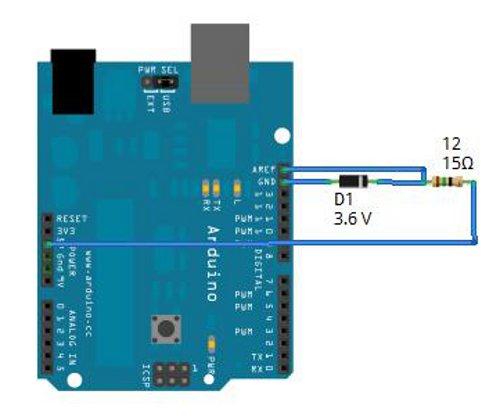Tutorial: Arduino and the AREF pin

Learn about the Arduino’s AREF pin and how to use it in this detailed tutorial.

[Updated 09/01/2013]

Today we are going to spend some time with the AREF pin – what it is, how it works and why you may want to use it. First of all, here it is on our boards:In chapter one of this series we used the analogRead() function to measure a voltage that fell between zero and five volts DC. In doing so, we used one of the six analog input pins. Each of these are connected to ADC (analog to digital conversion) pins in the Arduino’s microcontroller. And the analogRead() function returned a value that fell between 0 and 1023, relative to the input voltage.

But why is the result a value between 0~1023? This is due to the resolution of the ADC. The resolution (for this article) is the degree to which something can be represented numerically. The higher the resolution, the greater accuracy with which something can be represented. We call the 5V our reference voltage.

We measure resolution in the terms of the number of bits of resolution. For example, a 1-bit resolution would only allow two (two to the power of one) values – zero and one. A 2-bit resolution would allow four (two to the power of two) values – zero, one, two and three. If we tried to measure  a five volt range with a two-bit resolution, and the measured voltage was four volts, our ADC would return a value of 3 – as four volts falls between 3.75 and 5V. It is easier to imagine this with the following image:So with our example ADC with 2-bit resolution, it can only represent the voltage with four possible resulting values. If the input voltage falls between 0 and 1.25, the ADC returns 0; if the voltage falls between 1.25 and 2.5, the ADC returns a value of 1. And so on.

With our Arduino’s ADC range of 0~1023 – we have 1024 possible values – or 2 to the power of 10. So our Arduinos have an ADC with a 10-bit resolution. Not too shabby at all. If you divide 5 (volts) by 1024, the quotient is 0.00488 – so each step of the ADC represents 4.88 millivolts.

However – not all Arduino boards are created equally. Your default reference voltage of 5V is for Arduino Duemilanoves, Unos, Megas, Freetronics Elevens and others that have an MCU that is designed to run from 5V. If your Arduino board is designed for 3.3V, such as an Arduino Pro Mini-3.3 – your default reference voltage is 3.3V. So as always, check your board’s data sheet.

Note – if you’re powering your 5V board from USB, the default reference voltage will be a little less – check with a multimeter by measuring the potential across the 5V pin and GND. Then use the reading as your reference voltage.

What if we want to measure voltages between 0 and 2, or 0 and 4.6? How would the ADC know what is 100% of our voltage range?

And therein lies the reason for the AREF pin! AREF means Analogue REFerence. It allows us to feed the Arduino a reference voltage from an external power supply. For example, if we want to measure voltages with a maximum range of 3.3V, we would feed a nice smooth 3.3V into the AREF pin – perhaps from a voltage regulator IC. Then the each step of the ADC would represent 3.22 millivolts.

Interestingly enough, our Arduino boards already have some internal reference voltages to make use of. Boards with an ATmega328 microcontroller also have a 1.1V internal reference voltage. If you have a Mega (!), you also have available reference voltages of 1.1 and 2.56V. At the time of writing the lowest workable reference voltage would be 1.1V.

So how do we tell our Arduinos to use AREF? Simple. Use the function analogReference(type); in the following ways:

For Duemilanove and compatibles with ATmega328 microcontrollers:

• analogReference(INTERNAL); – selects the internal 1.1V reference voltage
• analogReference(EXTERNAL); – selects the voltage on the AREF pin (that must be between zero and five volts DC)
• And to return to the internal 5V reference voltage – use analogReference(DEFAULT);

If you have a Mega:

• analogReference(INTERNAL1V1); – selects the internal 1.1V reference voltage
• analogReference(INTERNAL2V56); – selects the internal 2.56V reference voltage
• analogReference(EXTERNAL); – selects the voltage on the AREF pin (that must be between zero and five volts DC)
• And to return to the internal 5V reference voltage – use analogReference(DEFAULT)

Note you must call analogReference() before using analogRead(); otherwise you will short the internal reference voltage to the AREF pin – possibly damaging your board. If unsure about your particular board, ask the supplier or perhaps in our Google Group.

Now that we understand the Arduino functions, let’s look at some ways to make a reference voltage. The most inexpensive method would be using resistors as a voltage divider. For example, to halve a voltage, use two identical resistors as such:For a thorough explanation on dividing voltage with resistors, please read this article. Try and use resistors with a low tolerance, such as 1%, otherwise your reference voltage may not be accurate enough. However this method is very cheap.

A more accurate method of generating a reference voltage is with a zener diode. Zener diodes are available in various breakdown voltages, and can be used very easily. Here is an example of using a 3.6V zener diode to generate a 3.6V reference voltage:For more information about zener (and other diodes) please read this article. Finally, you could also use a linear voltage regulator as mentioned earlier. Physically this would be the easiest and most accurate solution, however regulators are not available in such a wide range nor work with such low voltages (i.e. below 5V).

Finally, when developing your sketch with your new AREF voltage for analogRead();, don’t forget to take into account the mathematics of the operation. For example, if you have a reference voltage of 5V, divide it by 1024 to arrive at a value of 4.88 millivolts per analogRead() unit. Or as in the following example, if you have a reference voltage of 1.8V, dividing it by 1024 gives you 1.75 millivolts per analogRead() unit:

```// Example 22.1

int analoginput = 0; // our analog pin
int analogamount = 0; // stores incoming value
float percentage = 0; // used to store our percentage value
float voltage =0; // used to store voltage value

void setup()
{
Serial.begin(9600);
analogReference(EXTERNAL); // use AREF for reference voltage
}

void loop()
{
delay(200);
percentage=(analogamount/1024)*100;
voltage=analogamount*1.75; // in millivolts
Serial.print("Percentage of AREF: ");
Serial.println(percentage,2);
Serial.println(voltage,2);
}```

So if necessary, you can now reduce your voltage range for analog inputs and measure them effectively.Have fun and keep checking into tronixstuff.com. Why not follow things on twitterGoogle+, subscribe  for email updates or RSS using the links on the right-hand column, or join our Google Group – dedicated to the projects and related items on this website. Sign up – it’s free, helpful to each other –  and we can all learn something.

1.Jonathan says:

A very interesting article, as always! Keep up the good work 😛

•John Boxall says:

Hello
Thank you very much for your comment, I really appreciate it.
Cheers
john

2.Sonny says:

Maybe I’m not reading this right. But if you wanted 2.5v or 4.6v or whatever to be your max, wouldn’t you just use the ‘map” code to put the 5v ( or the 1024) into the range you desire? or am I not understanding the reasoning of doing this? Sorry in advance if this is really newbie thinking. Or for instance would this be useful if using your arduino as a volt meter, and changing the reference would change where the decimal point would be located?

•John Boxall says:

Hello Sonny
Yes, one could use map – but there is a possibility of loss with regards to mathematical rounding against using the required ‘real’ maximum voltage. Each to their own I guess 🙂
cheers
john

•follower says:

If you use software you will get worse resolution.

e.g. using map for 2.5V you would only get 512 possible values between 0V and 2.5V but set the AREF using hardware and you get 1024 possible values between 0V and 2.5V.

3.Mike says:

Wow thank you so very much for writing these tutorials! They are absolutely amazing!

•John Boxall says:

Hello Mike
Thank you very much for your comments, I really appreciate it – they make writing them more worthwhile
cheers
john

4.robodude666 says:

How accurate is the internal 1.1v reference voltage? I noticed that “5v” volts on the Arduino can jump around between 4.5 to 4.9v depending on if you’re using the DC jack or USB port, making the default reference not very predictable. This isn’t very good as you need to know the exact reference voltage to know how to weigh each digital value from an A/D conversion. A reference voltage of 4.69v gives a different digital value than a reference of 4.89v, etc.

If you needed superbly accurate A/D conversions, would it be better to get a 5v Low-dropout regulator that would be used to power the Arduino plus supply the AREF voltage to the Arduino?

•John Boxall says:

Hello
Good question. For reference voltages it is better to use say a 9V DC input, as there is not risk of dropout (or possible over-voltage) from the USB supply. However if one really needed a super accurate ADC, it would be better to use a dedicated IC (perhaps with an I2C interface).
cheers
john

5.cyril says:

I would like to use two analog inputs in my application. But I need to use the internal reference 1.1V for one analog input and external reference 4.6V for second analog input. Is it possible, pls?

•John Boxall says:

Hello
No, you can only use one per sketch.
John

•Jimmy says:

You could use the arduino to switch the aref pin to different value before analogReference() then read analog input one then switch back.

6.Ornskirk says:

What is the lowest reference voltage you could use?
Could I use 0.1v?

•John Boxall says:

Hello
Lowest possible AREF voltage is 1.1V
cheers
john

7.Brian Minielly says:

In item 5. above with the question about the two analogue inputs. I am confused by your response. Are you saying you can not use 2 analogue inputs? or is it that you can not use 2 different reference voltages in one sketch? I am building a solar system monitor and I want to poll voltages from several different places – the battery, the solar panel – but I would use the same reference voltage in each case. Will that work?
Brian

•John Boxall says:

Hello Brian
Thank you for your question. If you are using an identical reference voltage to measure two or more different places, that is OK. So your idea will work.
cheers
john

8.Mike says:

I’m a bit confused. I have to read an analog signal of 2 mV from a load cell. I had in mind to use a op-amp to amplify the voltage signal but this could be a valid solution. According to you, is it a valid alternative setting the AREF to 2V ?

•John Boxall says:

Sure you could use an op-amp, or the internal AREF of 1.1 – this would give you a resolution of ~1.07 mV

9.thermike says:

Thank you for your very interesting tutorial. I ‘m a bit confused though on how to use the external reference voltage i feed on aref pin and use this ref voltage in my scketch. Using back 4.88 v to multiply my analogRead(), doesn’t this mess my calculations? 5 / 1024 calculation uses a fixed 5v numerator, which might not be the case. I guess that max value will be AREF value. It is not implied here but should i feed the AREF pin voltage somewhere else as input? — thermike

•John Boxall says:

Hello
[You only need to leave comments once]
I’m not exactly sure how to interpret your question. If you had an AREF voltage of 4.88V, and analogRead() returned 5, that would represent ((5/1024)*4.88) or 23.8 millivolts.
cheers
john

•thermike says:

Hi,
OK, although you get the same result, I would say (AREF V/1024)*analogread=(4.88/1024)*5 since the parenthesis represent the steps (?). But how do you know the AREF voltage in your sketch?
I feed the half voltage of Vc, due to voltage divider to my AREF, as you describe above, and the Vc might fluctuate a bit. It might be 2.44v to 2.55v (I get this measurements with a multimeter). So I might be feeding AREF with variable V. I have to take into account this variation or not? Cheers

•John Boxall says:

Generally one would create a smoothed power supply of the required voltage for AREF, e.g. use an LM317T and smoothing capacitors. The the floating voltage will not be a problem.

10.Øsse says:

Hi,

I was wondering about the maximum ohm values that can be used in a voltage divider with resistors.
In an application supplied by a small battery this would be of interest, and the power draw by the resistors should be as low as possible.

Does the AREF pin have a defined (fixed?) input resistance?

Thanks,
Helge.

•John Boxall says:

Hello
To be honest I couldn’t really tell you. If you really want to minimise current use in the voltage divider try for precise resistors in the Mohm range.
John

11.nesdnuma says:

Hello John, this is an interesting tutorial but there is something I don’t understand. If I want to use the Uno’s internal 1.1V reference, should I only insert the analogReference(INTERNAL) code and use the analog inputs as usual only or should I also use the AREF pin ?

•John Boxall says:

Hello.
Yes, for internal no need for AREF pin.
john

•nesdnuma says:

OK, thanks John !

12.uGAga says:

Thanks a lot!

13.Jan S says:

Is there any way to set the reference to between two non-zero voltages? I have a sensor that outputs between 2.3 and 2.7 Volts – can i set the arduino uno to take the 1024 steps between those values instead?

•John Boxall says:

Sorry that isn’t possible using an Arduino.

14.isolatedsensor says:

You can use an isolated power supply at the sensor then ground the arduino to the 2.3 volt. Then use the arduino internal 1.1 volt Aref to accurately measure the .4 volt in reference to the 2.3 that will was the original 2.7. I hope that makes sense.

15.cruzerdog says:

John, great article. Googled this and found the best explained answer on the internet!!!

Gary
USA

•John Boxall says:

Cheers
john

16.Marcus says:

Hello,

I just read on arduino website that ” there is an internal 32K resistor on the AREF pin.” in this case then the voltage which we will get at AREF will not be exactly what we apply…in that case the aref will be 2.85mv rather then 3.22 mv. Can anyone suggest

17.Steve says:

what’s the value of the resistor to use with the zener? in the diagram it looks like 15Ohm (?) but that’s too low and it will result in about 85mA current draw and Aref voltage a little more than 3.6V. I think it should be in the 70-100Ohm area

18.Mike says:

Just found your site last night from the Arduino article on Wikipedia and I’m back today from a link on the Arduino blog. I can tell I’m going to spend a lot of time learning here. Thanks for sharing your knowledge!

19.Sindre says:

An interesting discovery: I messed around with the reference voltage, trying to get it as low as possible. I got it to 0.57 V on a programmable precision voltage source. Anything lower and the output yelds (1023) no matter what I feed the analog pin. So the smallest change I can measure is 0.57/2^10 = 0.57 mV. This is a bit too high, as I am potentially going to measure µV differences. I thought the only option for me to get any lower was to interface an external ADC or amplify my input signal.

However, I discovered that if I lower the clock speed, the reference voltage can be lowered further. I used both the hardware prescaler and the “clock_prescale_set(clock_div_64);”-command to lower the clock by a factor of 192 and was able to lower the reference voltage to 0.22 V, meaning a resolution with steps equal to 215 mV. Still isn’t enough though.

Could anyone confirm that lowering the clock speed leaves room for a lower AREF?

20.john wind says:

Sindre, is there an error in your math?. 0.22 v / 1023 = 215uV not 215mV

21.Kevin Houck says:

Hello,
So if I am using a breadboard arduino that takes it power from an existing device that runs on around 3v and I use the internal default voltage setting, will that internal reference voltage still be 5v, or will it just be the maximum vcc?

Thanks, and I can elaborate if this is a little unclear

•John Boxall says:

Internal reference Will be the voltage that you’re supplying the board.

22.anup says:

Hello I have etched PCB of Atmega168 and I am using analog pin as a output pin do I need to use AREF pin if required then please suggest me better solution ASAP

•John Boxall says:

If you’re using an analogue pin as an output you don’t need to use AREF with regards to that.

23.Ivan Aliaga says:

Percentage of AREF: 0.00
24.Stefan says: